Ex 2.3

Chapter 2 Class 11 Relations and Functions
Serial order wise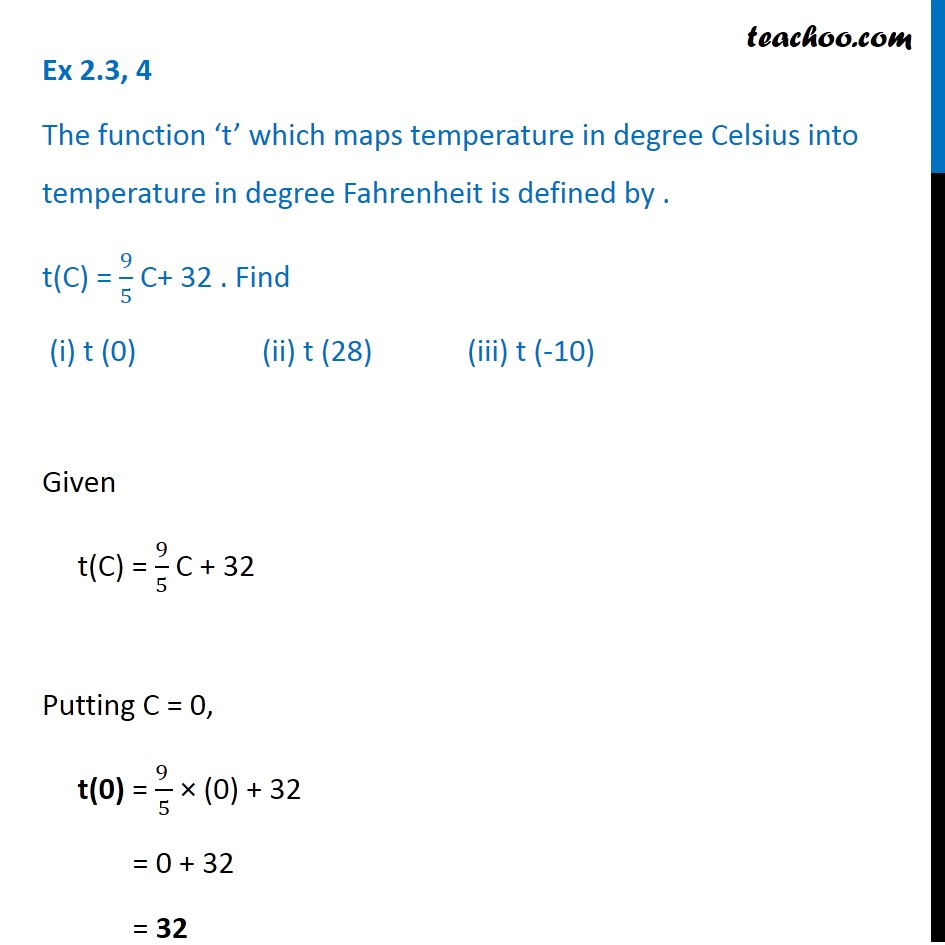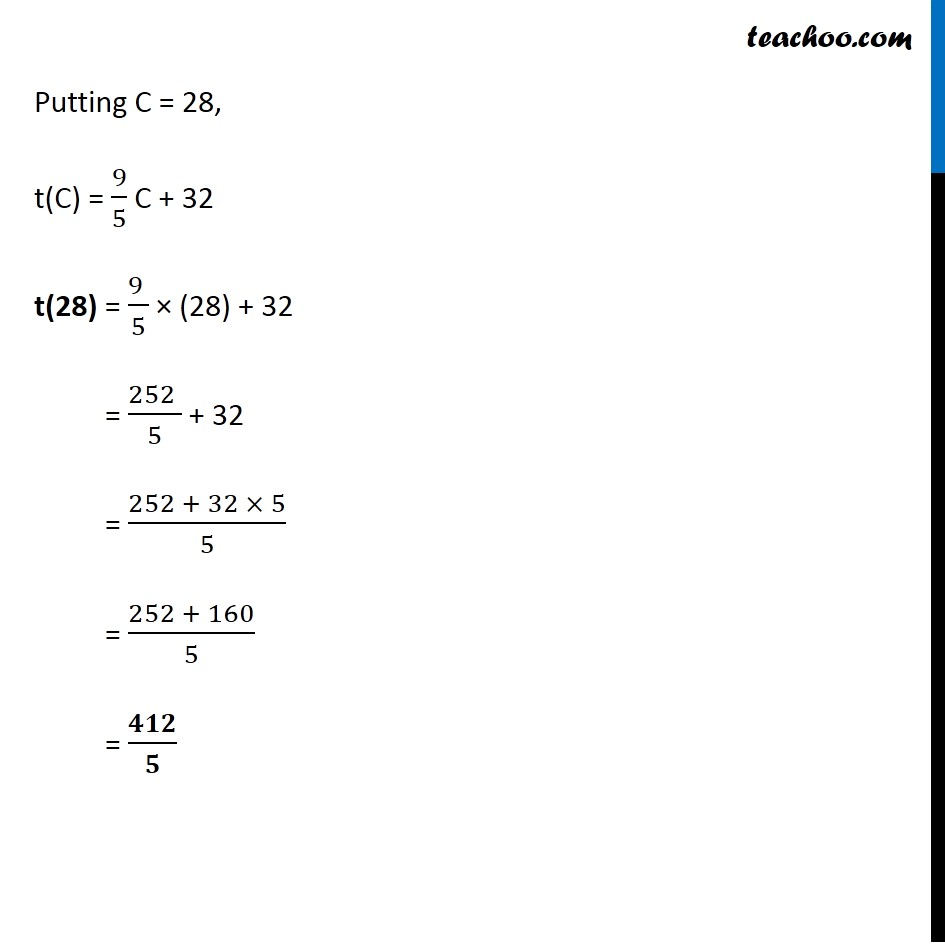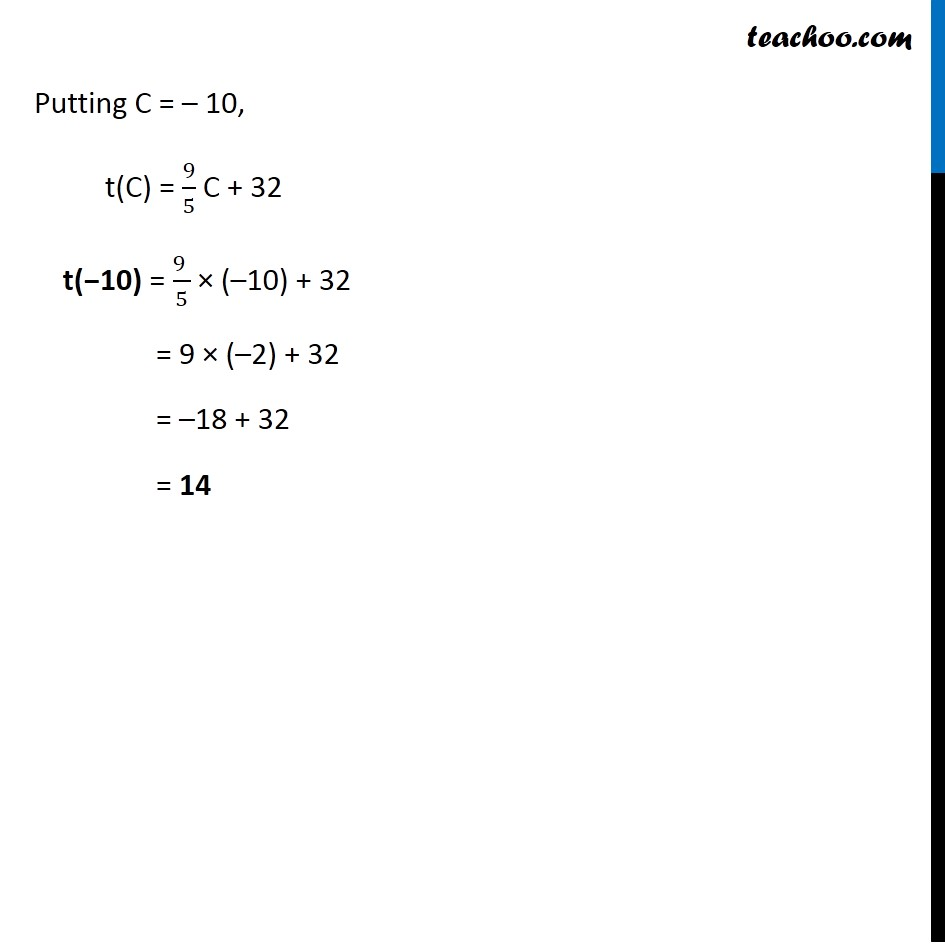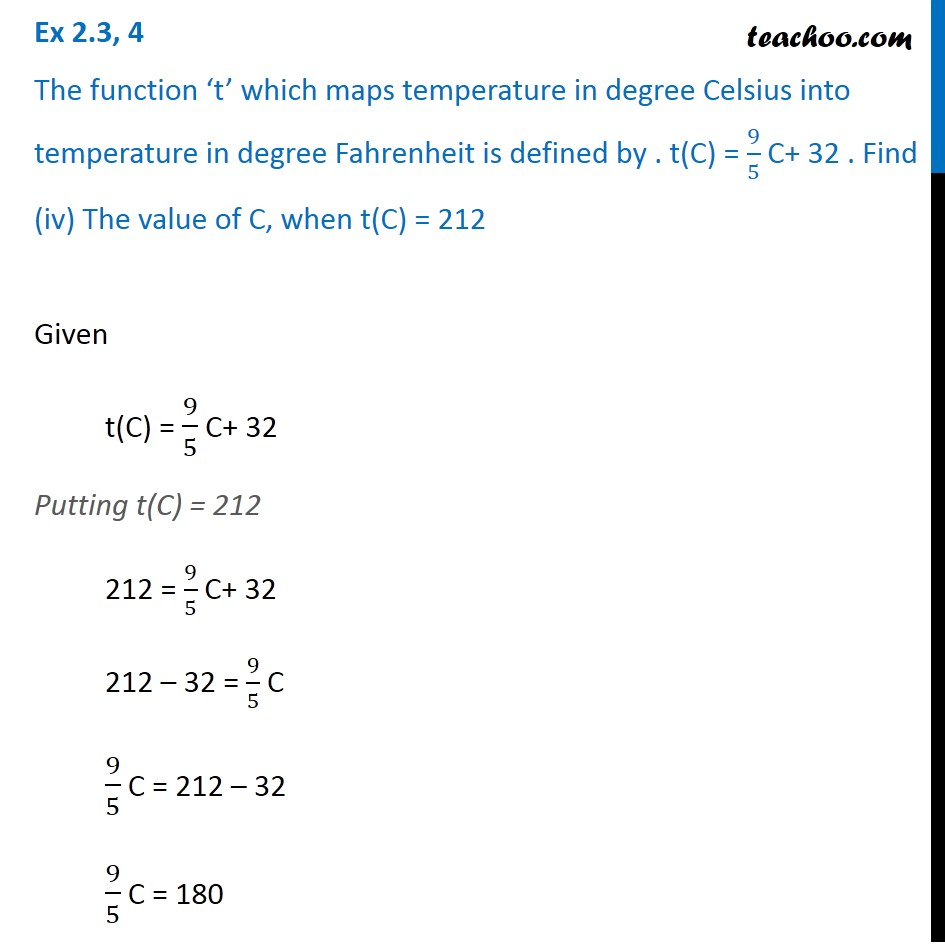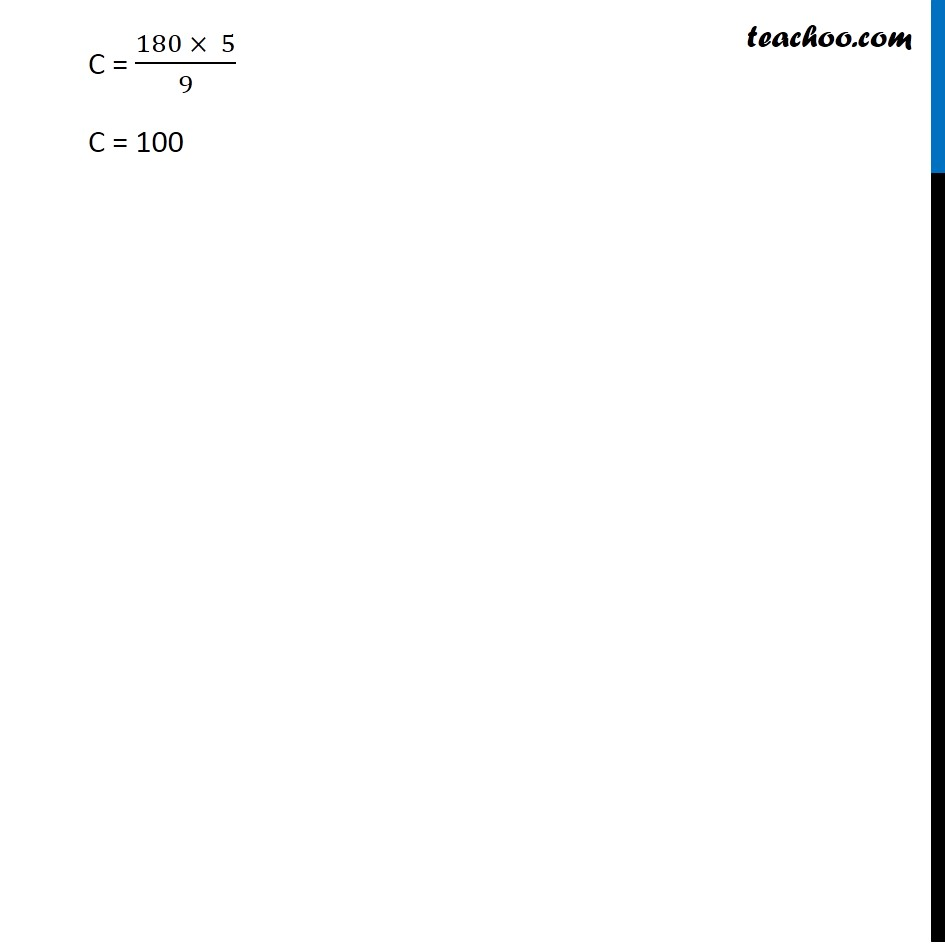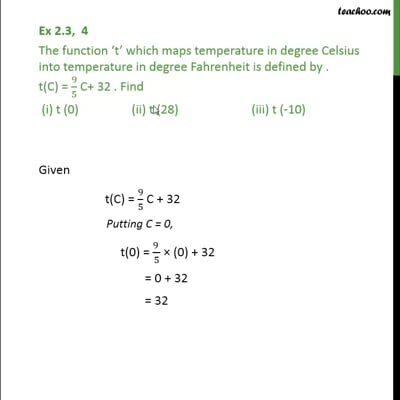This video is only available for Teachoo black users

Solve all your doubts with Teachoo Black (new monthly pack available now!)

### Transcript

Ex 2.3, 4 The function ‘t’ which maps temperature in degree Celsius into temperature in degree Fahrenheit is defined by . t(C) = 9/5 C+ 32 . Find (i) t (0) (ii) t (28) (iii) t (-10) Given t(C) = 9/5 C + 32 Putting C = 0, t(0) = (9 )/5 × (0) + 32 = 0 + 32 = 32 Putting C = 28, t(C) = 9/5 C + 32 t(28) = (9 )/5 × (28) + 32 = (252 )/5 + 32 = (252 + 32 × 5)/5 = (252 + 160)/5 = 𝟒𝟏𝟐/𝟓 Putting C = – 10, t(C) = 9/5 C + 32 t(−10) = (9 )/5 × (–10) + 32 = 9 × (–2) + 32 = –18 + 32 = 14 Ex 2.3, 4 The function ‘t’ which maps temperature in degree Celsius into temperature in degree Fahrenheit is defined by . t(C) = 9/5 C+ 32 . Find (iv) The value of C, when t(C) = 212 Given t(C) = 9/5 C+ 32 Putting t(C) = 212 212 = 9/5 C+ 32 212 – 32 = 9/5 C 9/5 C = 212 – 32 9/5 C = 180 C = (180 × 5)/9 C = 100